# Monte Carlo methods

• Recall, from earlier, that Monte Carlo methods refer to the idea of approximating an integral with an average of $$K$$ random variables, where $$K$$ is the number of iterations in our approximation algorithm.

$\frac{1}{K} \sum_{k=1}^K f(X_k) \approx \int f(x) \pi(x) {\text{d}}x$

• Today we introduce Markov chain Monte Carlo (MCMC), which is the most flexible way to do Monte Carlo.
• With MCMC, $$X_1, X_2, \dots$$ is the list of samples visited in a Markov chain
• With MCMC, the above approximation is justified using a law of large numbers for Markov chains

# Most common setup in Bayesian statistics

• Let $$x$$ denote the (vector of) latent (unobserved) variables
• Let $$y$$ denote the observations
• Let $$\gamma(x, y)$$ denote the joint distribution
• We will omit dependence on $$y$$ in the notation: $$\gamma(x) = \gamma(x, y)$$
• We assume that for a give hypothesized $$x$$, we can compute the numerical value $$\gamma(x)$$
• Let $$\pi(x | y)$$ denote the posterior
• Again, omit $$y$$ from the notation: $$\pi(x) = \pi(x | y)$$
• Hence: there is some $$C$$ such that $$\pi(x) = \gamma(x) / C$$
• Here $$C$$ does not depend on $$x$$
• It happens to be the marginal likelihood but we won’t use this fact today

# Extracting the essential ingredients of the “common setup”

• $$\pi(x)$$ is an “intractable distribution” called the target
• we seek to compute $$\int f(x) \pi(x) {\text{d}}x$$, where $$f$$ is a test function
• $$\pi(x) \propto \gamma(x) / C$$, where
• $$\gamma(x)$$ is the unnormalized density which can be “computed pointwise”
• $$C$$ is an unknown constant, the normalization constant, computing it is assumed intractable

# Outline

• Today we will go over one of the most powerful way to simulate the random variables suitable for Monte Carlo average: the Metropolis-Hastings algorithm
• Side note on pedagogic approach: I will use a new route to teach this material
• typically Metropolis Hastings (MH) is introduced by “guessing” a certain ratio called the MH ratio which is then shown to have a nice property (“invariance”); then one special case of this ratio is used to introduce a method called “slice sampling”
• here I will do the reverse: I will introduce “slice sampling” which is more intuitive and justify it from first principles, then derive MH as a consequence of slice sampling

# Prerequisite: a bit of Markov chain theory

Question: how to design a sampler for a uniform distribution on a set $$A$$?

Example: $$A = \{0, 1, 2\}$$

Idea: use a random walk so that it scales to complicated/high dimensional $$A$$

### Poll: what random walk has a uniform stationary distribution on $$A$$

1. Move to a neighbor at random
2. Move to a neighbor at random, except at the boundary, where you stop moving
3. Move to a neighbor at random, including yourself (self-transition)
4. None of these walks would work

# Markov chain 1 (incorrect)

Move to one neighbor at random?

How to check if this is correct:

1. Naive method: simulate a long chain, compute a histogram from the list of states visited
2. Exercise: explain why the code below is equivalent to the “Naive method”
compute_stationary_distribution <- function(matrix) {
eig <- eigen(t(M))
eigenvalues = eig$values i <- which.max(Re(eigenvalues[abs(Im(eigenvalues)) < 1e-6])) statio <- (eig$vectors)[,i]
statio <- statio / sum(statio)
return(statio)
}

M = matrix(c(
0,   1, 0,
1/2, 0, 1/2,
0,   1, 0
), nrow=3, ncol=3, byrow=T)

barplot(compute_stationary_distribution(M))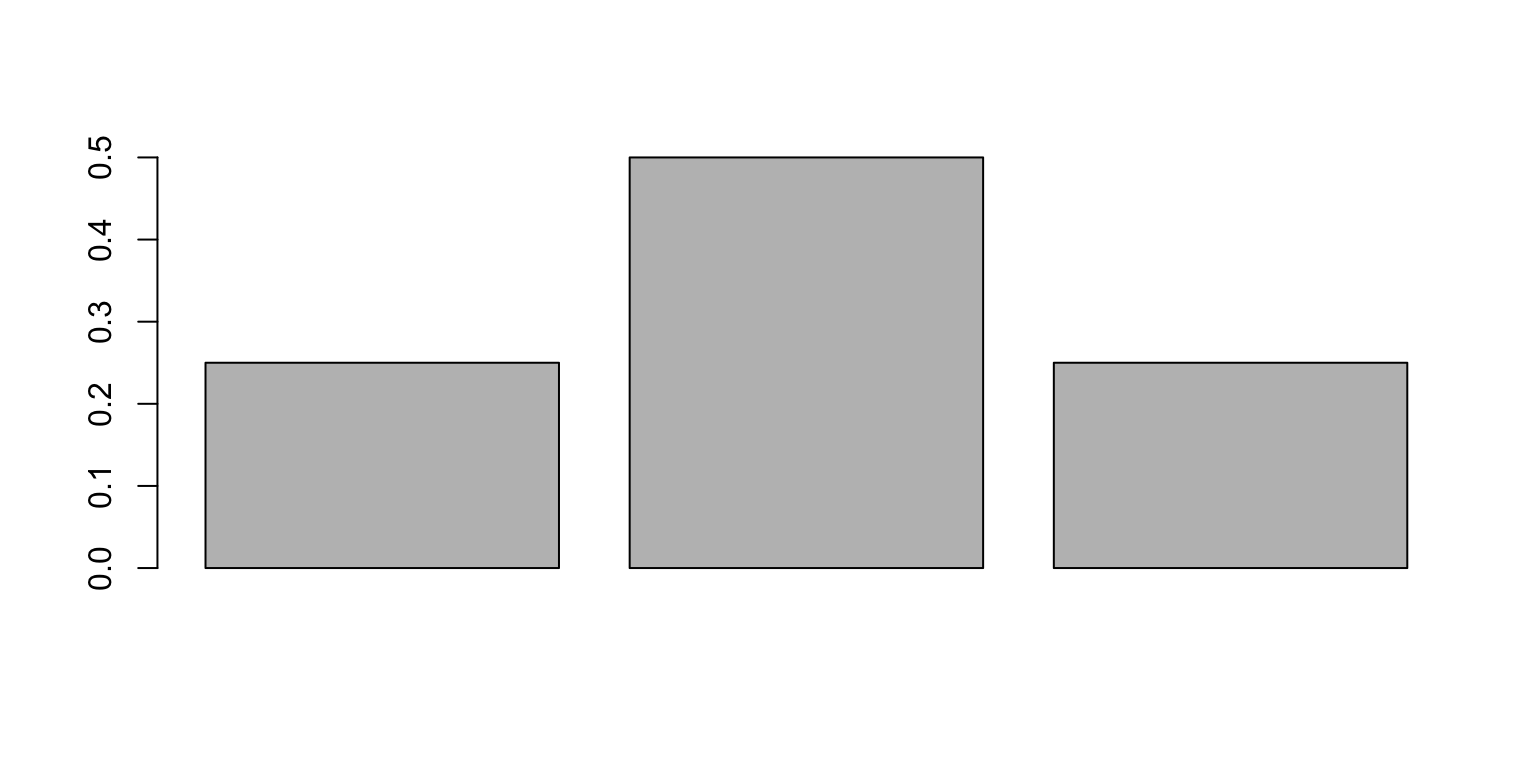# Markov chain 2 (incorrect)

Move to a neighbor at random, except at the boundary, where you stop moving.

No: you would get a random stationary distribution (either a Dirac delta at state 1 or a Dirac delta at state 3)

# Markov chain 3 (incorrect)

Move to a neighbor at random, including a self-transition

M = matrix(c(
1/2,   1/2, 0,
1/4, 1/2, 1/4,
0,   1/2, 1/2
), nrow=3, ncol=3, byrow=T)

barplot(compute_stationary_distribution(M))# Markov chain 4: propose + accept reject

• Say we are at position $$x_t \in A$$ at iteration $$t$$
• Propose to go to left or right with equal probability (an example of a proposal distribution $$q(x^* | x_t)$$).
• We get a proposal $$x^*$$
• However this proposal might be outside of $$A$$!
• Basic accept-reject step:
• If $$x^* \in A$$, set $$x_{t+1} = x^*$$ (accept)
• Else, set $$x_{t+1} = x_t$$ (reject)
M = matrix(c(
1/2,   1/2, 0,
1/2,   0,   1/2,
0,     1/2, 1/2
), nrow=3, ncol=3, byrow=T)

barplot(compute_stationary_distribution(M))# Understanding why idea/walk 1 fails while idea/walk 4 works

• Think of a very large population of people each with a laptop running MCMC
• There are 3 rooms corresponding to the 3 states $$A = \{0, 1, 2\}$$
• Depending on the state on their laptop, each person goes in the corresponding room

# Occupancy vector and transition matrix

• Room occupancies can be organized into a vector. Normalize by number of people and get a distribution.
• If you start with occupancies $$v$$ and let everyone run their MCMC for one step and do their room change, you get a new occupancy $$v'$$
• Exercise: convince yourself $$v' = v M$$ where row $$i$$ of $$M$$ is the transition probabilities for an MCMC at state $$i$$. I.e. $M_{i,j} = {\mathbb{P}}(X_{t+1} = j | X_t = i)$

# Stationarity

• The distribution of interest is $$\pi = (1/3, 1/3, 1/3)$$.
• Let us try to build $$M$$ such that if we start with an occupancy given by the distribution of interest, $$v = \pi$$, then after one step that occupancy is exactly preserved, $v' = \pi M \Rightarrow v' = \pi$ (this is called global balance)
• For ‘idea/walk 4’ this is easy to see: between any pair of rooms, $$i$$ and $$j$$ the number of people going from $$i$$ to $$j$$ is the same as the number going from $$j$$ to $$i$$ (this is an instance of detailed balance/reversibility, $$M_{i,j} \pi_i = M_{j,i} \pi_j$$)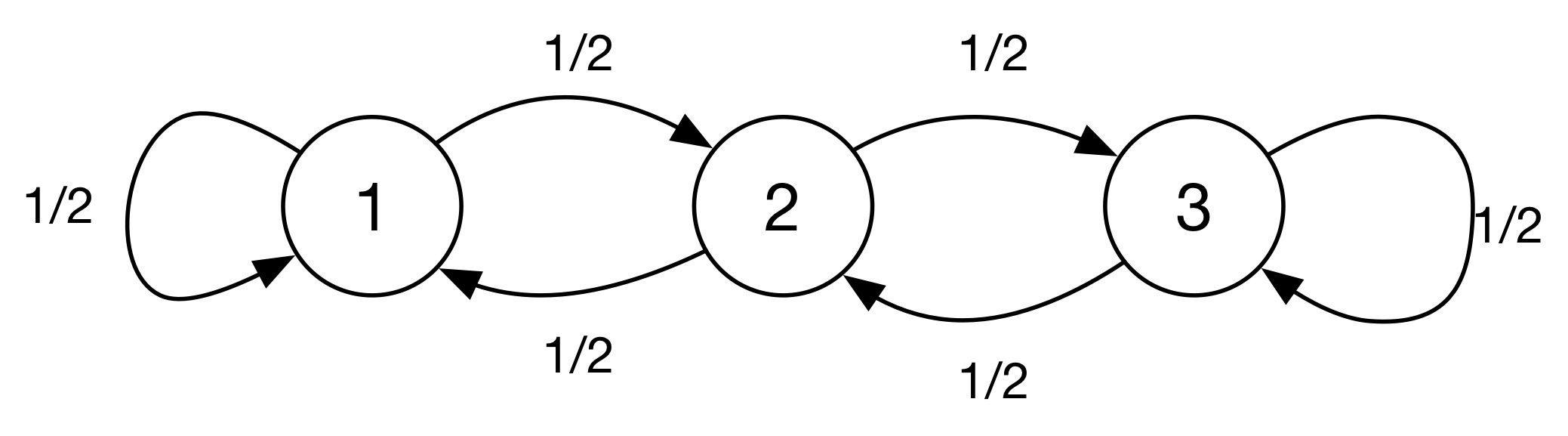• Compare with ‘idea/walk 1’ which does not satisfy global balance: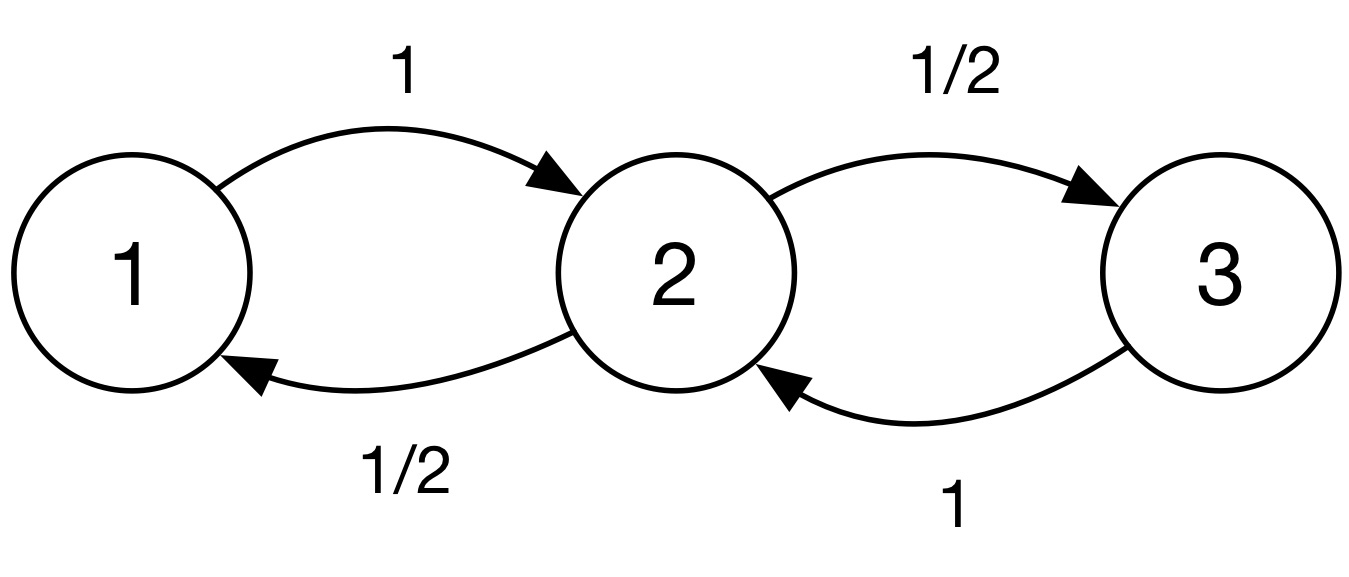• Exercises:
• show that detailed balance/reversibility implies global balance
• show that the other way around is not true (hint: consider for example a “cyclic tour”)

# Quick overview of (finite) Markov chain theory

• Law of Large Number: if we let $$X_n$$ denote which of the three state you visit at time $$n$$, for random walk 4, then $\frac{1}{n} \sum_{i=1}^n f(X_i) \to \frac{f(0) + f(1) + f(2)}{3} \;\;\text{(a.s.)}$

• Optional exercise: prove the LLN for Markov chain in this simplified setup.

• More generally: if a random walk on a discrete space can reach all states from any state (irreducibility), and satisfies global balance, then its sample average satisfies a law of large numbers.
• Do not need aperiodicity for LLN (if you don’t know what aperiodicity is, great! you won’t need it)
• Aperiodicity is a distraction from the MCMC point of view.
• In some sources you sometimes find it discussed in great lengths when the author has a fundamental misunderstanding of how MCMC works

# Generalization

• With a simple generalization of this argument, we now have an MCMC algorith for any uniform distribution on a “connected” set $$A$$ which could be in any space (continuous, discrete, mixed, etc).
• Condition that the proposal needs to satisfy in this first generalization: symmetric uniform proposal, $$q(x|x') = q(x'|x)$$ for all $$x, x' \in A$$,
• Exercise:
• Show the Markov chain induced by the above proposal is, for all $$x\in A$$ (edit: assume also $$q(x|x) = 0$$) $K(x'|x) = {{\bf 1}}[x' \neq x] {{\bf 1}}[x' \in A] q(x'|x) + {{\bf 1}}[x' = x] \sum_{x''} {{\bf 1}}[x'' \notin A] q(x''|x)$
• Use this to prove that $$K$$ satisfies detailed balance and hence global balance.
• Only looking at uniform distributions seems quite restrictive!
• But using a clever trick we will show this is actually all we need…

# Slice sampling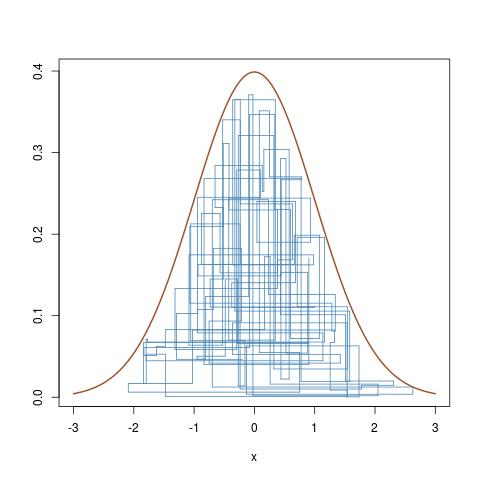• Recall the setup we are interested in: posterior density of the form: $\pi(x) = p(x|y) = \frac{\text{prior}(x)\times\text{likelihood}(y|x)}{p(y)} = \frac{\gamma(x)}{Z}$
• Idea: it is enough to do a uniform random walk under the graph of the function

• Notation:
• The walk is denoted $$(x_1, h_1), (x_2, h_2), (x_3, h_3), \dots, (x_n, h_n)$$
• $$x_i$$ is the state of interest (unknown parameter)
• $$h_i$$ is the height of the walk under the curve (just to help us sample, “auxiliary variable”)
• Recall: how to use the random walk to do Bayesian inference..
• How to compute sample mean: $$\frac{1}{n} \sum x_i$$
• How to compute credible interval?

# Slice sampling: some details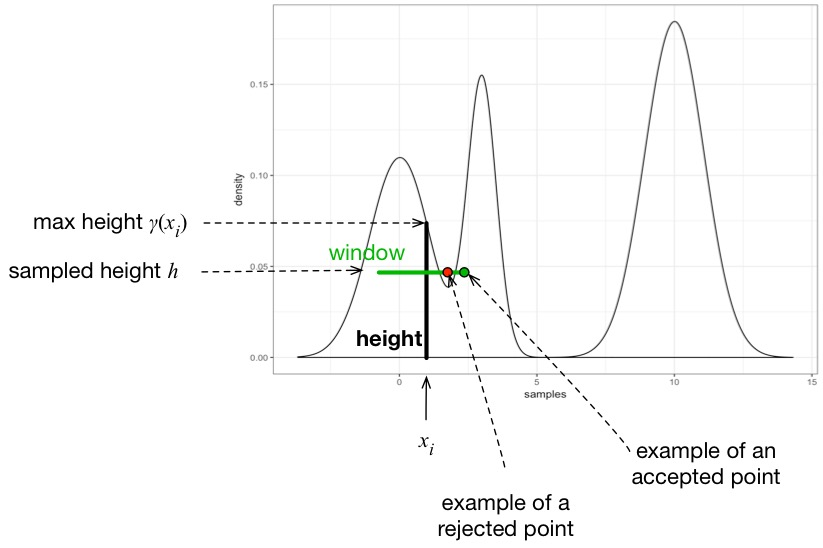• Propose a change for one variable at the time
• Look at current point $$x_i$$,
• compute a maximum height $$= \gamma(x_i)$$ (length of black bold line)
• sample height $$h$$ uniformly in $$[0, \gamma(x_i)]$$ (i.e. sample a point in the black bold line)
• Propose to move $$x^\star$$ uniformly in a window (green) around the current position, say $$x^\star$$ uniform in $$[x_i - 1, x_i + 1]$$
• These two steps define a proposed point $$(x^\star, h)$$. If this proposed is still under the density (example shown as green circle), accept, otherwise (example shown as red circle) reject and stay where you were

For more on slice sampling (in particular, how to avoid to be sensitive with respect to the size of the window size): see the original slice sampling paper by R. Neal

# Relation with Metropolis-Hastings (MH)

• The slice sampler proposes alternates between these two steps:
• Sample new $$x$$ position, $$x*$$
• Sample a height $$h$$ uniformly from 0 to the max height
• Metropolis-Hastings: combine the above two moves and replace the uniform height by a coin flip
• How? Let’s consider two cases

Case 1: proposing to go “uphill”, $$\gamma(x^*) \ge \gamma(x)$$. In this case, we always accept! See the picture: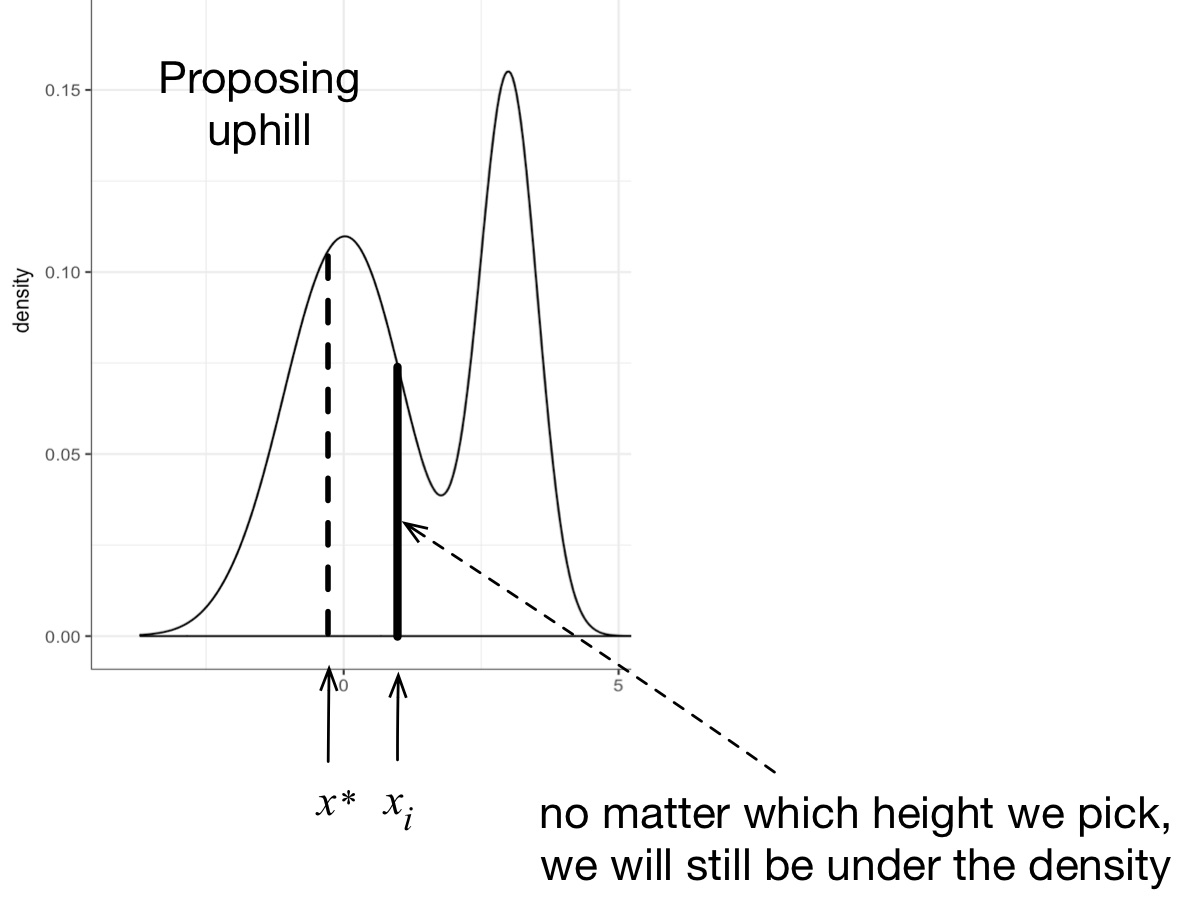Case 2: proposing to go “downhill”, $$\gamma(x^*) < \gamma(x)$$. In this case, we may accept or reject… See the picture: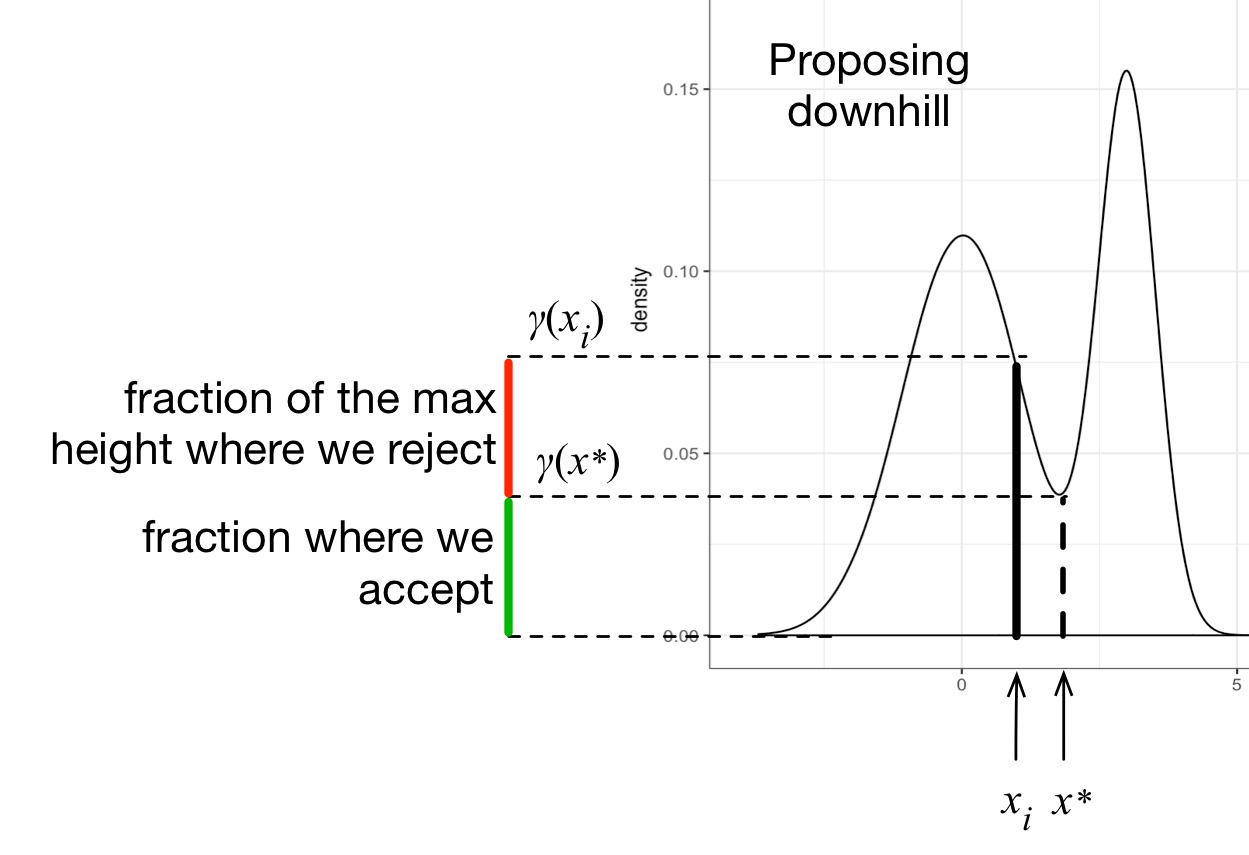### Poll: what is the probability that we will accept in case 2

1. $$\gamma(x_i)/\gamma(x^*)$$
2. $$\gamma(x^*)/\gamma(x_i)$$
3. $$\gamma(x^*) + \gamma(x_i)$$
4. $$\gamma(x^*) \cdot \gamma(x_i)$$
5. None of the above

# Acceptance probability

We can compute the probability that we accept! It’s the height of the green stick in bold divided by the height of the black stick in bold:$\frac{\gamma(x^*)}{\gamma(x_i)}$

This quantity is called the Metropolis ratio

# A special case of Metropolis algorithm

We obtain the following algorithm. Suppose the current state is $$x_i$$:

1. Propose a new position, $$x^*$$, uniformly in a ball around $$x_i$$
2. Compute the Metropolis ratio $r = \frac{\gamma(x^*)}{\gamma(x_i)}$
3. Simulate an acceptance variable $a \sim \text{Bern}(\min\{1, r\})$
• If $$a = 1$$: set $$x_{i+1} = x^*$$
• Else, set $$x_{i+1} = x_i$$

# General Metropolis algorithm

More general proposals:

• Instead of using a uniform ball, the same algorithm works (i.e. still admit a law of large numbers) if we generalize the first step into:
1. Propose a new position $$x^*$$, from a symmetric proposal density $$q(x^*|x_i)$$
• Here symmetric means $$q(x'|x) = q(x|x')$$; examples:
• Uniform ball
• Normal distribution centered around the current point

Combinations of proposals:

• Suppose you have two proposals $$q_1$$ and $$q_2$$
• The following algorithm also works (“alternation of Metropolis kernels”):
1. Do one iteration of the Metropolis algorithm with $$q_1$$
2. Do one iteration of the Metropolis algorithm with $$q_2$$
3. Go to 1.

# Another generalization: Metropolis-Hastings

• Suppose you want to use a proposal $$q(x'|x)$$ which is not symmetric.
• Then you need to change the Metropolis into the Metropolis-Hastings ratio: $\frac{\gamma(x^*)}{\gamma(x_i)} \frac{q(x_i|x^*)}{q(x^*|x_i)}$

• Proof sketch:
• Can be viewed as Metropolis on an augmented space
• Add an auxiliary variable, i.e. pretend we are sample a higher-dimensional distribution $$\tilde \pi(x, z) = \pi(x) q(z | x)$$ (note $$\pi(x)$$ is a marginal of $$\tilde \pi(x, z)$$)
• Consider the alternation of the two Metropolis kernels
1. $$q_1$$: sample $$z' \sim q(z | x)$$, keep $$x$$ unchanged
2. $$q_2$$: flip $$x$$ and $$z$$, i.e. $$x' = z$$, $$z' = x$$
• Exercises:
• Implementation:
• Write MH from scratch for the beta binomial problem (not in a PPL this time)
• Describe and implement a strategy to check that your implementation is correct
• Introduce a bug intentionally and make sure that your strategy above would have caught it
• Theory: use the steps below to complete the above argument to show MH admits $$\pi$$ as an invariant distribution (i.e. that its Markov kernel satisfies global balance with respect to $$\pi$$)
• Show the proposal $$q_2$$ is symmetric
• You can assume that the kernel corresponding to $$q_1$$ satisfies global balance (this is done with a Metropolis-within-Gibbs argument if you are curious)
• Show that these two steps combined lead to the Metropolis-Hastings ratio shown above